Back to Contents

# When Things Go Wrong

Some of the functions we have written so far have had a single, correct answer for each possible argument. For example, there’s no number we cannot halve. However, when we use more complicated types such as lists, there are plenty of functions which do not always have an answer – a list might not have a head or a tail, for example. Our `take` and `drop` functions were unsatisfactory in case of invalid arguments. For example, `take 3 [’a’]` would simply return `[]`. This is bad practice – we are hiding errors rather than confronting them.

OCaml has a mechanism for reporting such run-time errors (these are quite different from the type errors OCaml reports when it refuses to accept a program at all). This mechanism is exceptions.

There are some built-in exceptions in OCaml. For example `Division_by_zero`, which is raised when a program tries to divide a number by zero:

` OCaml`

`# 10 / 0;;`
`Exception: Division_by_zero.`

In order to signal bad arguments in functions like `take` and `drop`, we can rewrite them using the built-in exception `Invalid_argument`, which also carries a message written between double quotation marks. Typically we use this to record the name of the function which failed. The following program shows `take` and `drop` rewritten to use the `Invalid_argument` exception using `raise`. Note that these functions deal with two problems of our previous versions: a negative argument, and being asked to take or drop more than the number of elements in the list.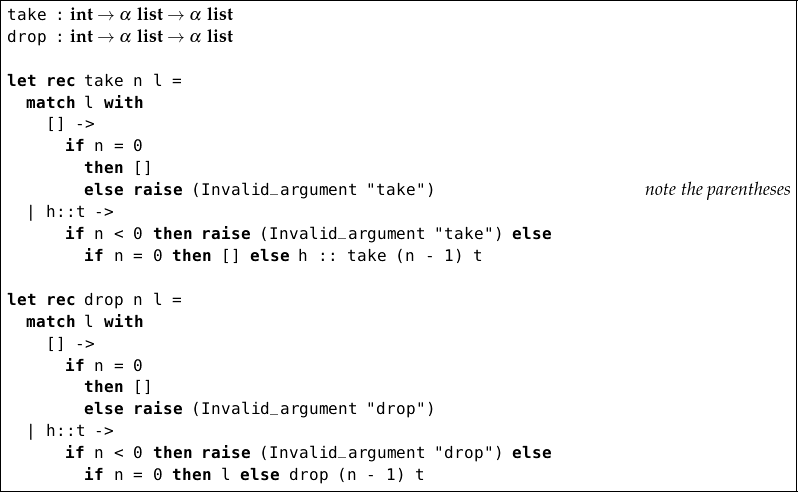We can define our own exceptions, using `exception`. They can carry information along with them, of a type we choose:

` OCaml`

`# exception Problem;;`
`exception Problem`
`# exception NotPrime of int;;`
`exception NotPrime of int`

We have defined two exceptions – `Problem`, and `NotPrime` which carries an integer along with it. Exceptions must start with a capital letter. The `of` construct can be used to introduce the type of information which travels along with an exception. Once they are defined we may use them in our own functions, using `raise`:

` OCaml`

`# exception Problem;;`
`exception Problem`
`# let f x = if x < 0 then raise Problem else 100 / x;;`
`val f : int -> int = <fun>`
`# f 5`
`- : int = 20`
`# f (-1);;`
`Exception: Problem.`

Exceptions can be handled as well as raised. An exception handler deals with an exception raised by an expression. Exception handlers are written using the `try` … `with` construct: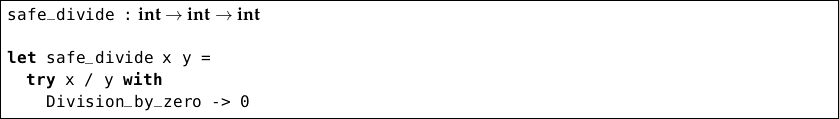The `safe_divide` function tries to divide `x` by `y`, but if the expression `x / y` raises the built-in exception `Division_by_zero`, instead we return zero. Thus, our `safe_divide` function succeeds for every argument.

How do the types work here? The expression `x / y` has type int and so the expression we substitute in case of `Division_by_zero` must have the same type: int, which indeed it does. And so, our rule that each expression must have one and only one type is not violated – `safe_divide` always returns an int.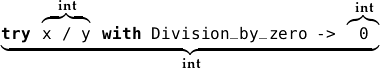Here is another example. The function `last` returns the last element of a list: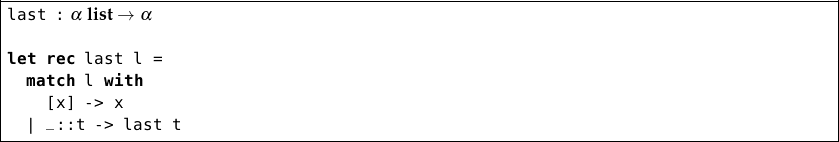The pattern match is incomplete, so whilst OCaml accepts the program it can fail at run-time. We can tidy up the situation by raising the built-in exception `Not_found`: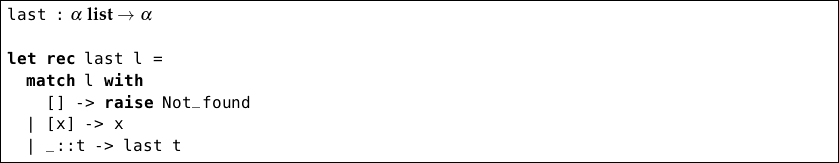The type of a function gives no indication of what exceptions it might raise or handle; it is the responsibility of the programmer to ensure that exceptions which should be handled always are – this is an area in which the type system cannot help us. Later in this book, we will see some alternatives to exceptions for occasions when they are likely to be frequently raised, allowing the type system to make sure we have dealt with each possible circumstance.

## Questions

1. Write a function `smallest` which returns the smallest positive element of a list of integers. If there is no positive element, it should raise the built-in `Not_found` exception.

2. Write another function `smallest_or_zero` which uses the `smallest` function but if `Not_found` is raised, returns zero.

3. Write an exception definition and a function which calculates the largest integer smaller than or equal to the square root of a given integer. If the argument is negative, the exception should be raised.

4. Write another function which uses the previous one, but handles the exception, and simply returns zero when a suitable integer cannot be found.

5. Comment on the merits and demerits of exceptions as a method for dealing with exceptional situations, in contrast to returning a special value to indicate an error (such as `-1` for a function normally returning a positive number).